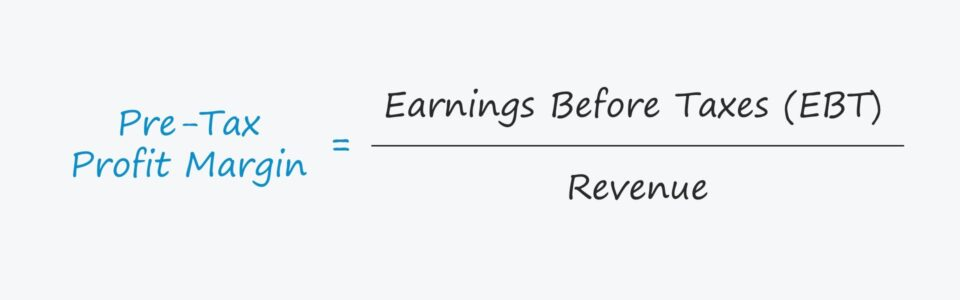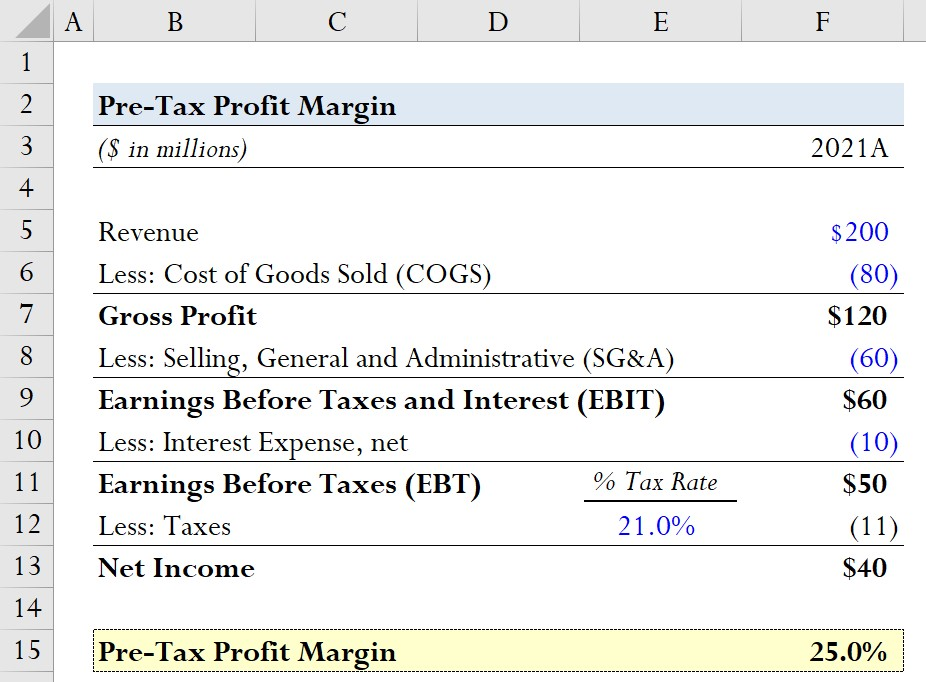Welcome to Wall Street Prep! Use code at checkout for 15% off.# Pre-Tax Profit Margin

Guide to Understanding the Pre-Tax Profit Margin## How to Calculate Pre-Tax Profit Margin?

The pre-tax profit margin ratio compares a company’s earnings before taxes (EBT) to its revenue in the corresponding period.

EBT, also known as “pre-tax income”, represents the residual earnings after operating expenses and non-operating expenses are accounted for, except taxes.

On the income statement, EBT represents a company’s taxable income for book purposes and is the final line item before taxes are deducted to arrive at net income (i.e. the “bottom line”).

## Pre-Tax Profit Margin Formula

The pre-tax profit margin (or EBT margin) is the percentage of profits retained by a company prior to fulfilling its required tax obligations to the state and federal government.

The pre-tax margin formula is calculated by dividing a company’s earnings before taxes (EBT) by its revenue.

Pre-Tax Profit Margin = Earnings Before Taxes (EBT) ÷ Revenue

Since profit margins are expressed in percentage form, the resulting amount from the formula above must subsequently be multiplied by 100.

The pre-tax profit margin answers the following question, “How much in earnings before taxes (EBT) is retained by a company per dollar of revenue generated?”

For example, a pre-tax margin of 40% means that for each dollar of revenue, a company’s EBT is \$0.40.

## What is a Good Pre-Tax Margin?

The earnings before taxes (EBT) profit metric excludes taxes – as implied by the name – making comparisons among industry peers more practical by removing the distorting effects from different tax structures and operating in different jurisdictions.

Based on the geographic location, a company’s corporate tax rate, and the state tax rate can differ substantially.

Further, a company can possess items such as unused tax credits and net operating losses (NOLs) that can impact its effective tax rate, which can cause its taxes to diverge from that of comparable companies.

One limitation of the pre-tax margin is that the metric is still affected by discretionary financing decisions, i.e. the capital structure of the company.

The decisions surrounding how to fund operations and the proportion of equity or debt that comprise the total capitalization are discretionary (and can skew financial results).

In particular, the interest expense could be higher for a company more reliant on debt financing than its industry peers. In this case, the company’s net income and net profit margin could be relatively lower than its peers, yet the underlying reason is related to its capital structure, not its operations.

Therefore, the operating margin and EBITDA margin are still the most widely used profitability margins, as those metrics are independent of both financing decisions and tax differences.

## Pre-Tax Profit Margin Calculator

We’ll now move to a modeling exercise, which you can access by filling out the form below.Submitting...

## Pre-Tax Profit Margin Calculation Example

Suppose we’re tasked with calculating the pre-tax profit margin of a company with the following financials for the fiscal year 2021.

• Revenue = \$200 million
• Less: Cost of Goods Sold (COGS) = (\$80) million
• Gross Profit = \$120 million
• Less: Selling, General and Administrative (SG&A) = (\$60) million
• Earnings Before Taxes and Interest (EBIT) = \$60 million
• Less: Interest Expense, net = (\$10) million
• Earnings Before Taxes (EBT) = \$50 million
• Less: Taxes @ 21% Tax Rate = (\$11) million
• Net Income = \$40 million

The two inputs we need to calculate the pre-tax margin are the earnings before taxes (EBT) and the revenue for 2021.

• EBT = \$50 million
• Revenue = \$200 million

Using the proper formula, our hypothetical company’s pre-tax profit margin comes out to be 25%.

• Pre-Tax Margin = \$50 million ÷ \$200 million = 25.0%

The 25% pre-tax margin implies that for each dollar of revenue generated, a quarter of it will remain at the earnings before taxes (EBT) line.Step-by-Step Online Course

### Everything You Need To Master Financial Modeling

Enroll in The Premium Package: Learn Financial Statement Modeling, DCF, M&A, LBO and Comps. The same training program used at top investment banks.